# How to Calculate and Solve for Gravitational Acceleration | Gravity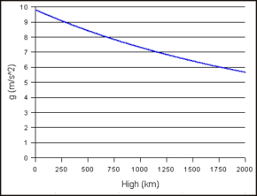The image above represents gravitational acceleration.

To compute for gravitational acceleration, three essential parameters are needed and these parameters are Gravitational Constant (G), Mass of the Earth (mEand Radius of the Earth (R).

The formula for calculating gravitational acceleration:

a = GmE /

Where:

a = Gravitational Acceleration
G = Gravitational Constant
mE = Mass of the Earth
R = Radius of the Earth

Let’s solve an example;
Find the Gravitational acceleration when the gravitational constant is 6.67E-11, the mass of the earth is 12 and the radius of the earth is 21.

This implies that;

G = Gravitational Constant = 6.67E-11
mE = Mass of the Earth = 12
R = Radius of the Earth = 21

a = GmE /
a = (6.67e-11)(12) / (21)²
a = 8.004e-10 / 441
a = 1.81e-12

Therefore, the gravitational acceleration is 1.81e-12 m/s.

Calculating Mass of the Earth when the Gravitational Acceleration, the Gravitational Constant and the Radius of the Earth is Given.

mE = a x R² / G

Where;

mE = Mass of the Earth
a = Gravitational Acceleration
G = Gravitational Constant
R = Radius of the Earth

Let’s solve an example;
Find the mass of the earth when the gravitational acceleration is 25, the gravtitaional constant is 6.67E-11 and the radius of the earth is 2.

This implies that;

a = Gravitational Acceleration = 25
G = Gravitational Constant = 6.67E-11
R = Radius of the Earth = 2

mE = a x R² / G
mE = 25 x 2² / 6.67E-11
mE = 25 x 4 / 6.67E-11
mE = 100 / 6.67E-11
mE = 14.99e+10

Therefore, the mass of the earth is 14.99e+10 m.

Nickzom Calculator – The Calculator Encyclopedia is capable of calculating the gravitational acceleration.

To get the answer and workings of the gravitational acceleration using the Nickzom Calculator – The Calculator Encyclopedia. First, you need to obtain the app.

You can get this app via any of these means:

To get access to the professional version via web, you need to register and subscribe for NGN 2,000 per annum to have utter access to all functionalities.
You can also try the demo version via https://www.nickzom.org/calculator

Apple (Paid) – https://itunes.apple.com/us/app/nickzom-calculator/id1331162702?mt=8
Once, you have obtained the calculator encyclopedia app, proceed to the Calculator Map, then click on Geology under Add-on.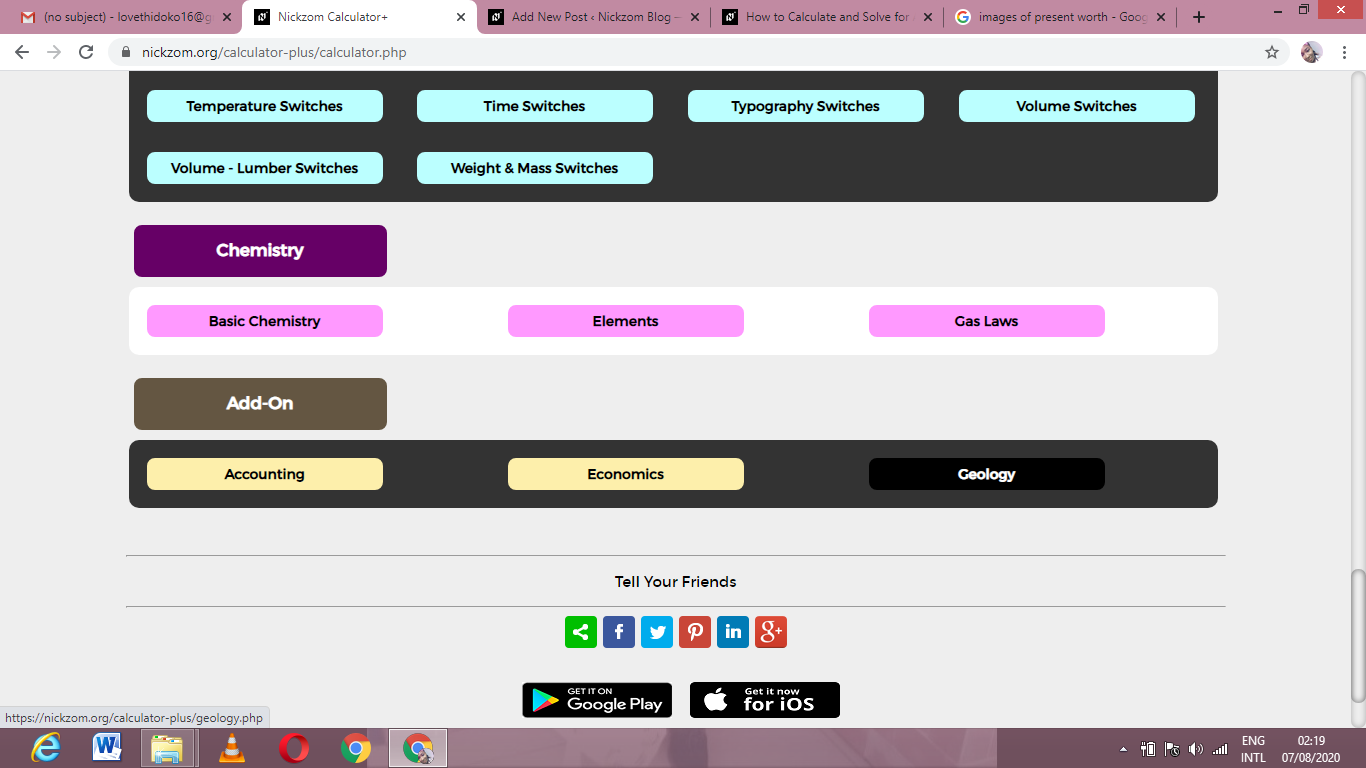Now, Click on Gravity under Geology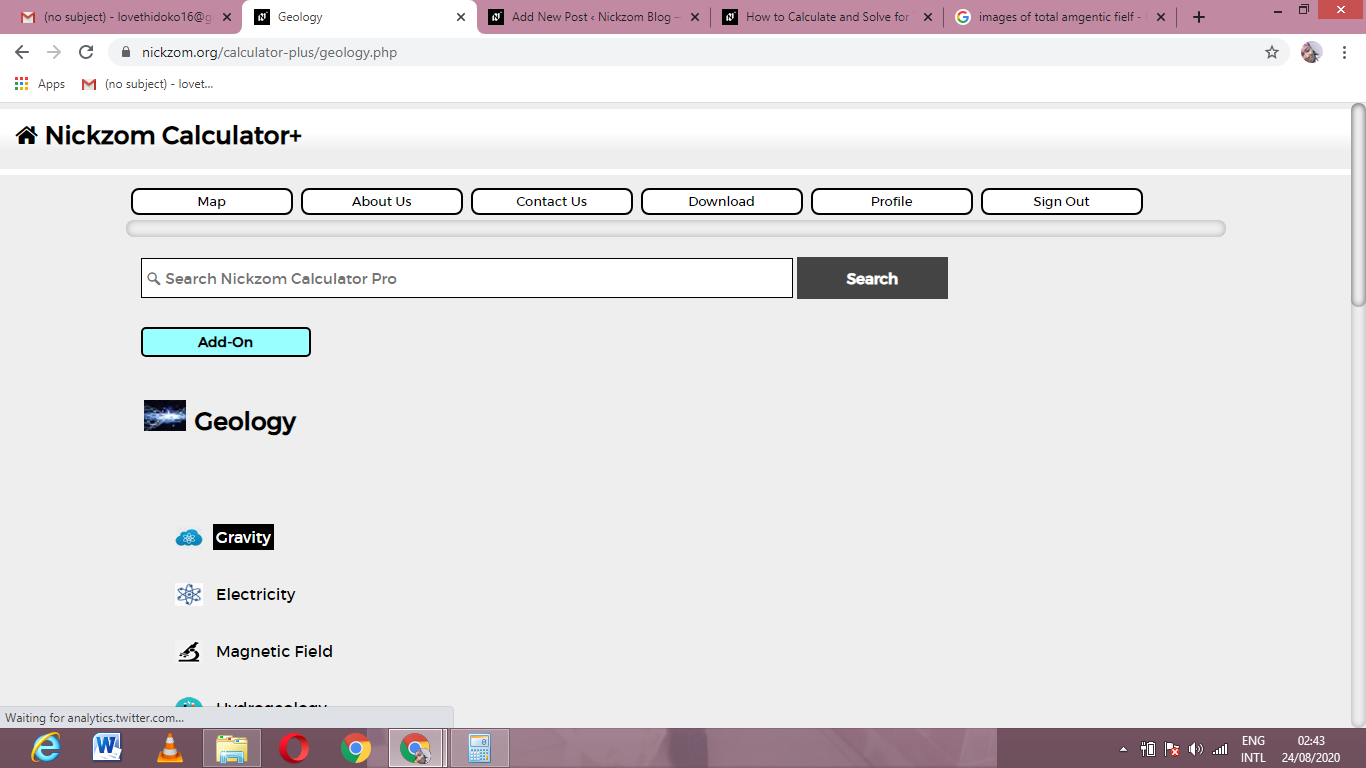Now, Click on Gravitational Acceleration under Gravity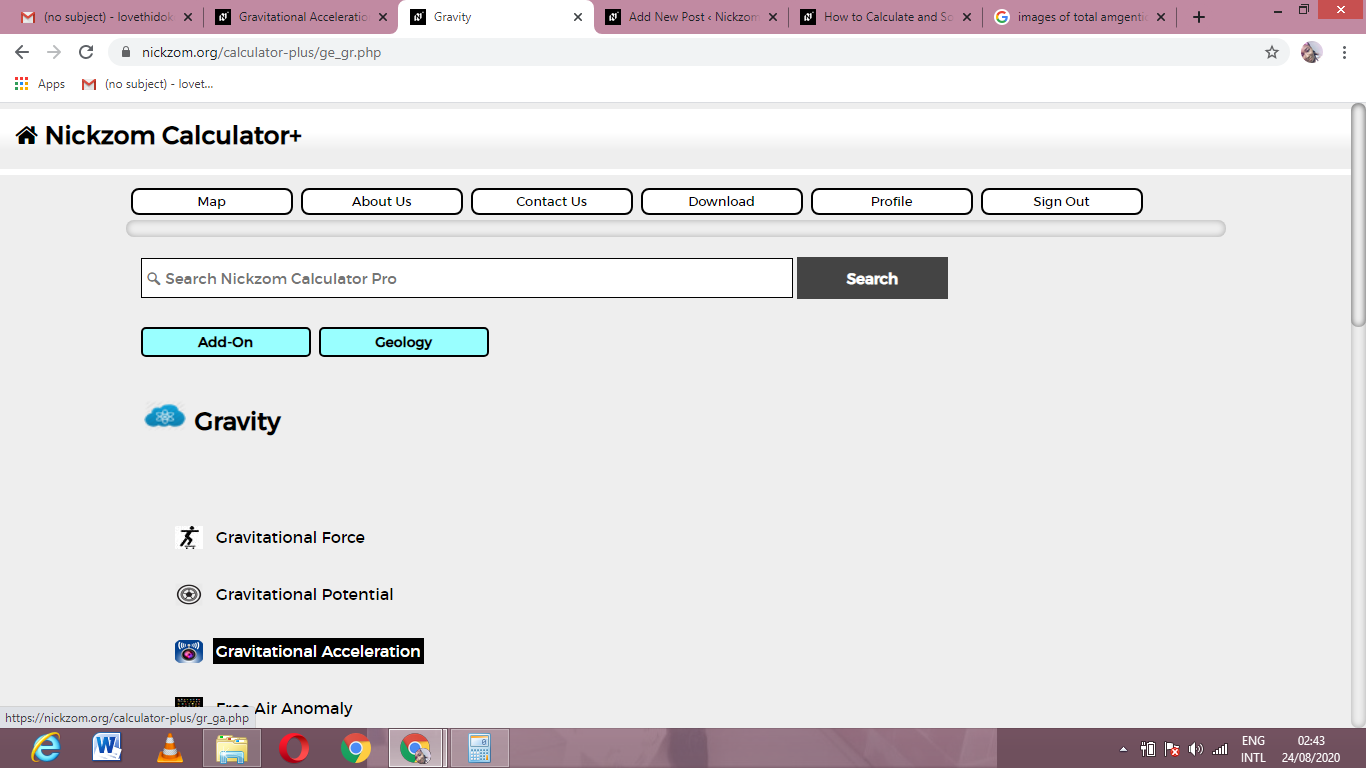The screenshot below displays the page or activity to enter your values, to get the answer for the gravitational acceleration according to the respective parameters which are the Gravitational Constant (G), Mass of the Earth (mEand Radius of the Earth (R).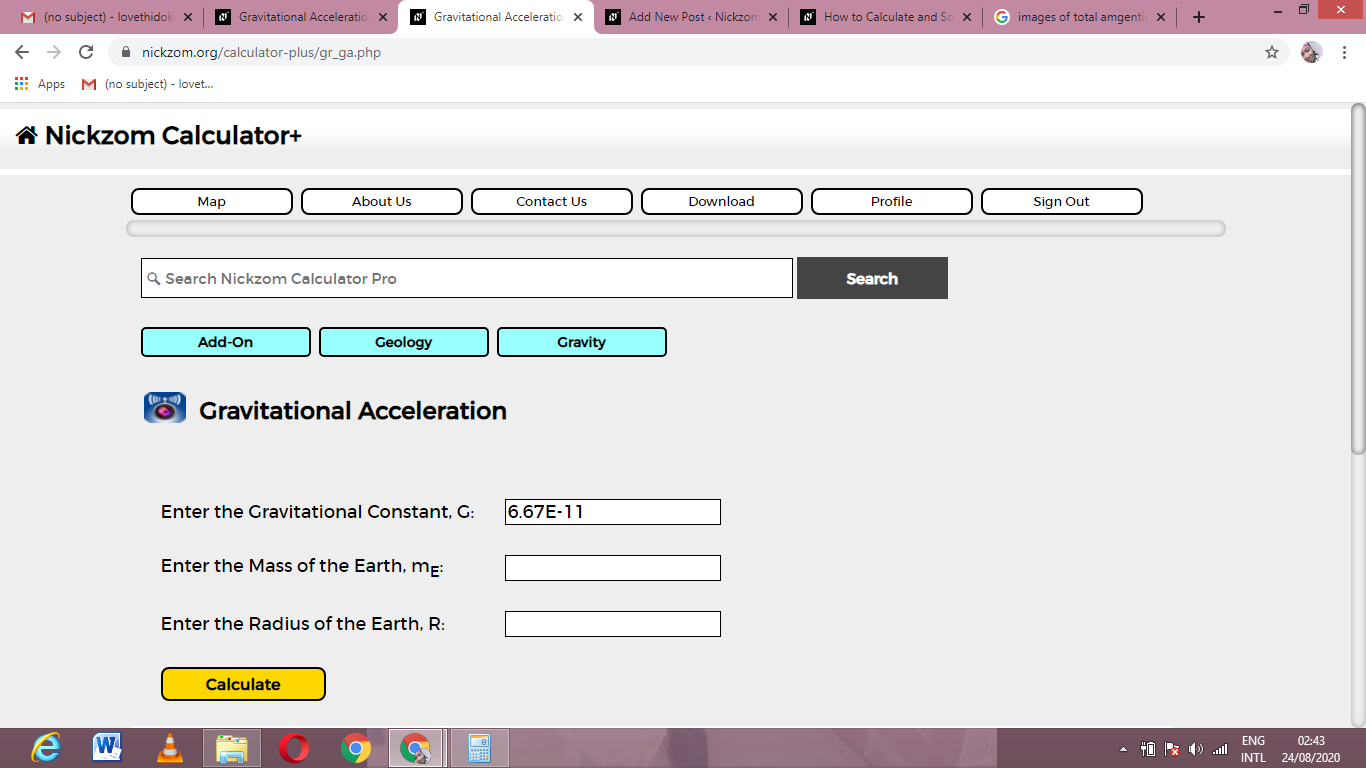Now, enter the values appropriately and accordingly for the parameters as required by the Gravitational Constant (G), Mass of the Earth (mEand Radius of the Earth (R).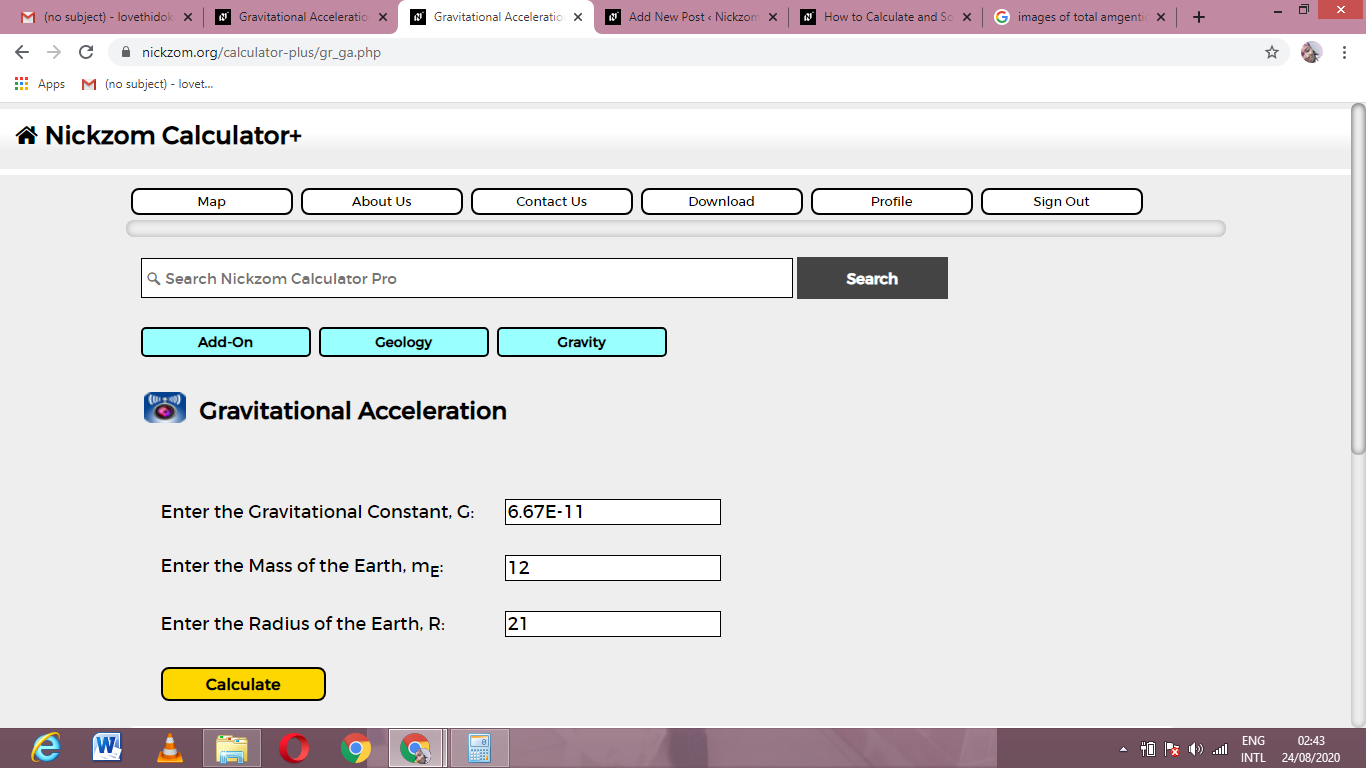Finally, Click on Calculate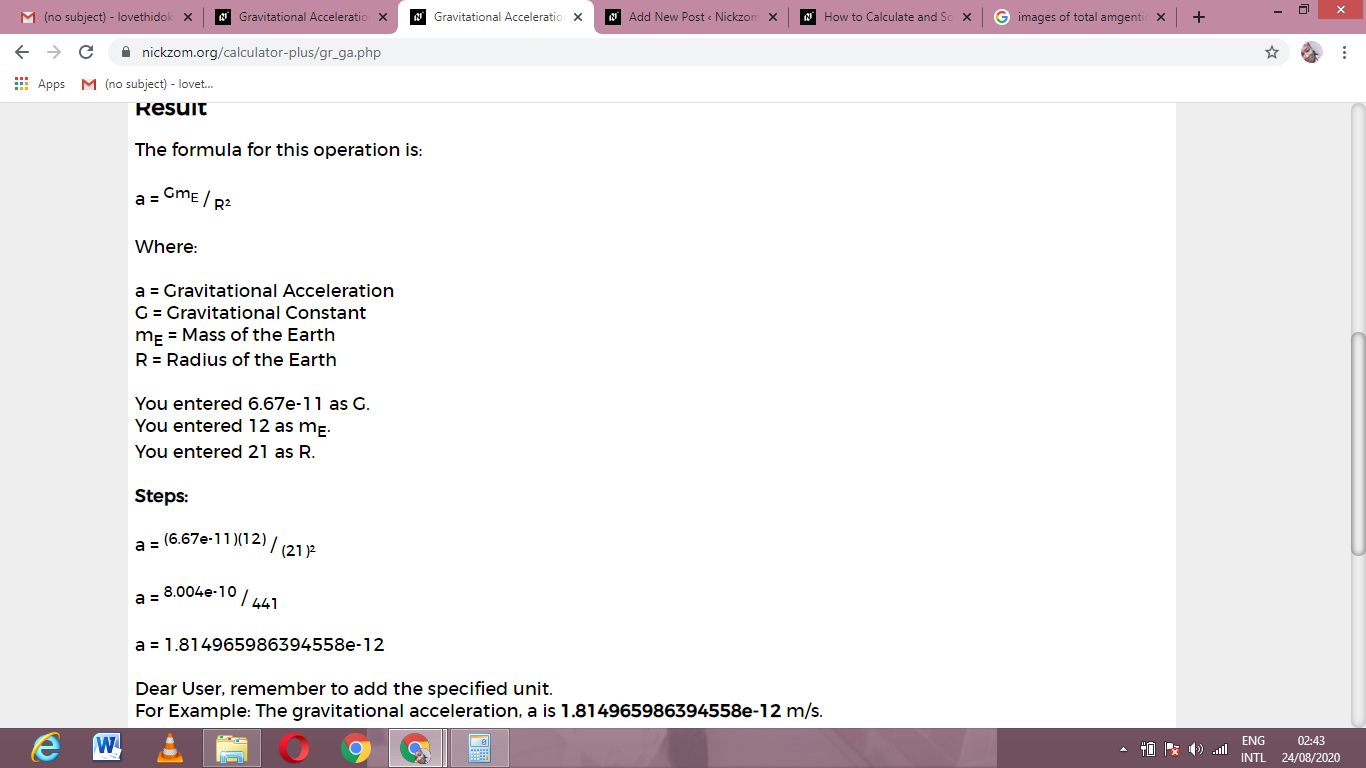As you can see from the screenshot above, Nickzom Calculator– The Calculator Encyclopedia solves for the gravitational acceleration and presents the formula, workings and steps too.